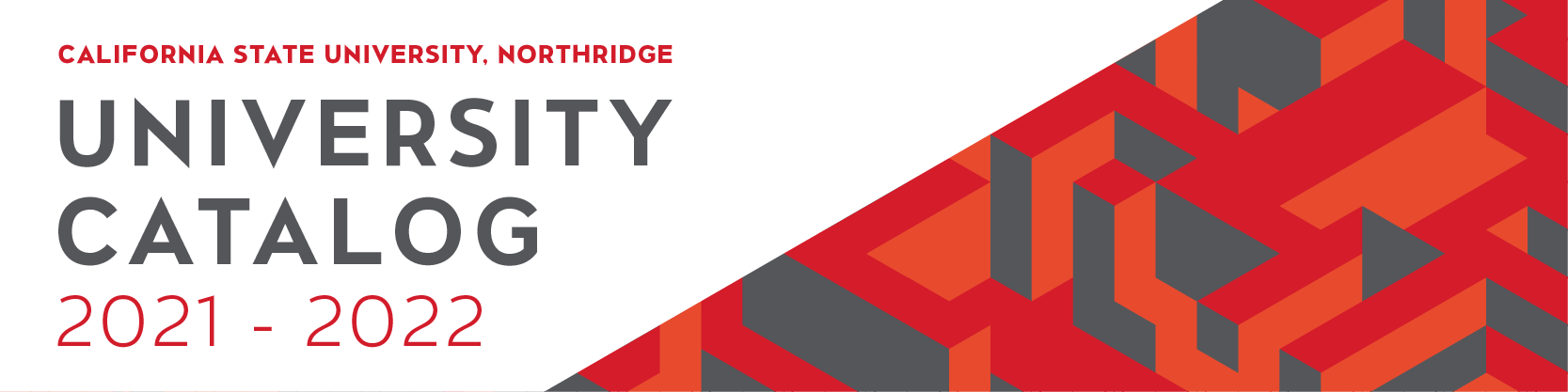## Course: MATH 483. Mathematical Modeling (3)

Prerequisites: Completion of MATH 340 and MATH 351 with a grade of “C” or better. Applications of mathematical techniques to solve selected problems in ecology, biology, economics, finance, social sciences, life sciences, physical sciences and engineering. Models discussed include deterministic, stochastic, optimization, static and dynamic ones. Emphasis is placed on the initial phase of building mathematical models and the final phase of interpreting the solutions in terms of real-life applications.

### MATH 483

Class NumberLocationDayTime

### MATH 483

Class NumberLocationDayTime